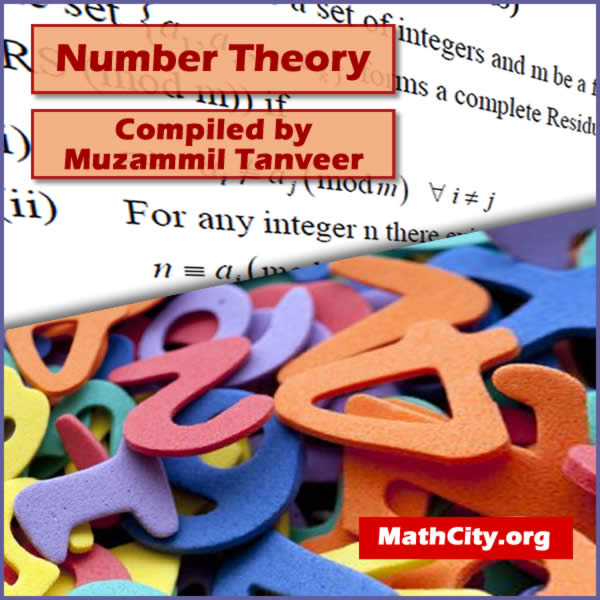# Number Theory by Dr Muhammad Umer ShuaibA subfield of mathematics called number theory studies the characteristics of positive integers. Higher arithmetic is another name for it. The study of the relationships between various types of numbers, including prime numbers, rational numbers, and algebraic integers, is done using number theory, one of the oldest fields of mathematics.

These notes are provided and composed by Mr. Muzammil Tanveer. We are really very thankful to him for providing these notes and appreciates his effort to publish these notes on MathCity.org

 Name Number Theory Dr. Muhammad Umer Shuaib Mr. Muzammil Tanveer 71 pages PDF (see Software section for PDF Reader) 593 kB
• Integer
• Divisibility
• Common Divisor
• Greatest Common Divisor
• Uniqueness
• Co-prime integer or Relatively prime integer
• Common Multiple
• Least common multiple (LCM)
• Linear Indeterminate equations or Linear Diophantine equations
• Congruence
• Complete Residue System (CRS)
• Reduced Residue System (RRS)
• Euler $\phi$ function
• Prime Number
• Composite Number
• Perfect Number
• Twin Primes
• Prime Triplet
• Powerful integer
• Fermat numbers

Please click on View Online to see inside the PDF.

• notes/number-theory-umer-shuaib